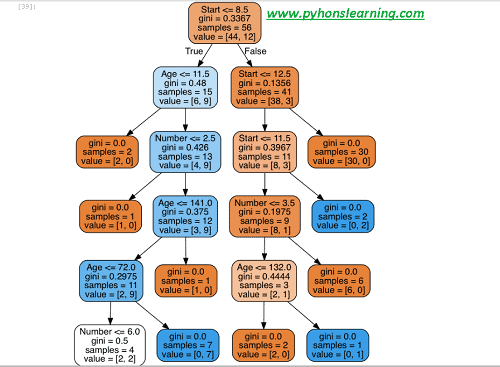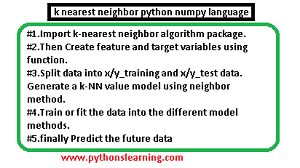## which is best python modules for machine learning

A module is a file in Python which organizes code in a systematic manner in order to increase our understanding and accessibility of the code. This code mostly consists of…Python Program to Construct and Implement binary search tree python 3.8

## Python Program to Construct and Implement binary search tree python 3.8

Tree is a type of DataStructure, consisting mainly of nodes. Every node has some child node until we reach the last node having no childrens. Those last nodes are called…

## Prediction Using Supervised Machine Learning Algorithms step by step

Introduction : Hey guys, in this article i am share my one internship Machine learning project code, which is Prediction using Supervised Machine Learning Algorithms. so let's see : Prediction…

## k means clustering in python using iris dataset

k means clustering algorithm python example K Means Clustering is unsupervised learning algorithm in python (i.e.it's tries to cluster the different data based on their similarity) and another meaning is…

# Decision Trees and Random Forests classifier in Python

Welcome everyone, Today we will see Decision Trees and Random Forests classifier-and Types in Python so let’s start:

In this project following steps are used to performed operation:
• Import algorithm Decision Trees and Random Forests classifier package.
• Get the data
• Split data into x/y_training and x/y_test data.
• Train or fit the data into the different model methods.
• Prediction and Evaluation the data
• Decision Trees visualization
• Random Forests
• finally generate the Tree (learn different python terminologies)

## Import algorithm Decision Trees and Random Forests classifier package

`Import Librariesimport pandas as pdimport numpy as npimport matplotlib.pyplot as pltimport seaborn as sns%matplotlib inline`

Get the Data

`df = pd.read_csv('Decision Trees.csv')`

`Decision  Age Number Start0 present 34 3 91 absent 58 4 152 absent 28 5 83 present 72 3 44 absent 81 4 15`

### Split data into x/y_training and x/y_test data.

Let’s start to split up the data into a training and test set.

`from sklearn.model_selection import train_test_splitx = df.drop('Decision Trees',axis=1)y = df['Decision Trees']x_train, x_test, y_train, y_test = train_test_split(x, y, test_size=0.20)`

#### check out Decision Trees

We will start just by training a single decision trees in this section.

`from sklearn.tree import DecisionTreeClassifierdtree = DecisionTreeClassifier()dtree.fit(x_train,y_train)`
`DecisionTreeClassifier(class_weight=None, criterion='gini', max_depth=None,            max_features=None, max_leaf_nodes=None, min_samples_leaf=1,            min_samples_split=2, min_weight_fraction_leaf=0.0,            presort=False, random_state=None, splitter='best')`

How to Prediction and Evaluation the data
Let’s start to evaluate our decision tree.

`predictions = dtree.predict(x_test)from sklearn.metrics import classification_report,confusion_matrixprint(classification_report(y_test,predictions))`

`         precisionx    recallx  f1-scorex   supportx    present       0.80      0.80      0.80        15    absent       0.45      0.45      0.45         10avg / total       0.75      0.75      0.75        25`

`print(confusion_matrix(y_test,predictions))[[18  4] [ 2  3]`

Tree Visualization

`from IPython.display import Image  from sklearn.externals.six import StringIO  from sklearn.tree import export_graphvizimport pydot `

`features = list(df.columns[1:])features['Ages', 'Numbers', 'Starts']dot_data = StringIO()  export_graphviz(dtree, out_file=dot_data,feature_names=features,filled=True,rounded=True)graph = pydot.graph_from_dot_data(dot_data.getvalue())  Image(graph.create_png())  `Decision Trees and Random Forests classifier-Types in Python

# k nearest neighbor python numpy language:

Welcome everyone in python crash course (Machine learning). This is first part of this section,if you want to learn SVM in python then click on it.
K Nearest Neighbors method also used for data prediction purpose, so in his section we will learn  K Nearest Neighbors predict method.k nearest neighbor python numpy language

## How do you use K nearest neighbor in Python language in smart way?

In this project following steps are used to performed operation:
• Import knearest neighbor algorithm package.
• Then Create feature and target variables using function.
• Split data into x/y_training and x/y_test data.
• Generate a kNN value model using neighbor method.
• Train or fit the data into the different model methods.
• finally Predict the future data

### What is K nearest neighbor used for?

suppose you have been given a classified data set from a any popular company,they give you the data and the target classes and say predicts a class for a new data point based off of the features.
Let’s do it!

#### k nearest neighbor python 3.10

Import Libraries
import pandas as pd
import seaborn as sns
import matplotlib.pyplot as plt
import numpy as np
%matplotlib inline

Get the Data
Set index_col=0

df = pd.read_csv(“Sample Classified Data”,index_col=0)

WTS PTS EQS SBS LQS QWS FDS PJS HQS NXS TARGETC CLASSES
0.923917 1.172073 0.467946 0.655464 0.880862 0.252608 0.659697 0.343798 0.979422 1.231409 1
0.645632 1.033722 0.545342 0.865645 0.934109 0.658450 0.675334 1.213546 0.681552 1.492702 1
0.5521360 1.201493 0.921990 0.8775595 1.526629 0.720781 1.776351 1.154483 0.957877 1.285597 0
1.434204 1.386726 0.653046 0.425624 1.142504 0.875128 1.509708 1.380003 1.522692 1.253093 0
1.579491 0.949750 0.627280 0.768976 1.232537 0.703727 1.815596 0.646691 1.463812 1.519167 1

Standardize the Variables  K Nearest Neighbors method
In the next step we drop the TARGETC CLASSES from the data because this type of data affect on the prediction.

from sklearn.preprocessing import StandardScaler
scaler = StandardScaler()
scaler.fit(df.drop(‘TARGETC CLASSES’,axis=1))
StandardScaler(copy=True, with_mean=True, with_std=True)
scaled_features = scaler.transform(df.drop(‘TARGETC CLASSES’,axis=1))
df_feat = pd.DataFrame(scaled_features,columns=df.columns[:-1])

WTS  PTS EQS SBS LQS QWS FDS PJS HQS NXS
-0.045232 0.185907 -0.913431 0.3229629 -1.033637 -2.308375 -0.798951 -1.482368 -0.949719 -0.643314
-1.674836 -0.430348 -1.025313 0.625388 -0.444847 -1.152706 -1.129797 -0.202240 -1.828051 0.636759
-0.9988702 0.339318 0.301511 0.785873 2.031693 -0.870156 2.5109818 0.285707 -0.682494 -0.377850
0.992841 1.060193 -0.621399 0.635299 0.452820 -0.267220 1.770208 1.066491 1.241325 -1.026987
1.149275 -0.640392 -0.709819 -0.557175 0.822886 -0.936773 0.6996782 -1.472352 1.040772

Train Test Split method:

from sklearn.model_selection import train_test_split
X_train, X_test, y_train, y_test = train_test_split(scaled_features,df[‘TARGETC CLASSES’],
test_size=0.20)

Using KNN method
Remember we will start with k=1 value.

from sklearn.neighbors import KNeighborsClassifier
knn = KNeighborsClassifier(n_neighbors=1)
knn.fit(X_train,y_train)
KNeighborsClassifier(algorithm=’auto’, leaf_size=20, metric=’minkowski’,
metric_params=None, n_jobs=1, n_neighbors=1, p=2,
weights=’uniform’)
pred = knn.predict(X_test)

Predictions and Evaluations using knn
Let’s start to evaluate our KNN model!

from sklearn.metrics import classification_report,confusion_matrix

print(confusions_matrix(y_test,pred))

[[124  19]
[ 12 145]]

print(classification_report(y_test,pred))

precisionx    recallx  f1-scorex   supportx
1       0.92      0.87      0.89       144
0       0.99      0.92      0.90       158
avg / total       0.94      0.90      0.89       300

Choosing a correct value for K for this
we use the elbow method to pick a correct K Value:

error_rate = []

# Advantages and disadvantages of Machine Learning :

Welcome everyone, In this article we will learn which is advantages and disadvantages of Machine Learning ? First, we will talk about the Advantages of Machine Learning. so Let’s start:advantages and disadvantages in machine learning

## Advantages of Machine Learning

• Efficient Handling of Data
• Best for Education and Online Shopping
• Continuous Improvement
• Automation for everything
• Automation of Everything
• Wide Range of Applications
• Trends and patterns identification
• Scope of Improvement
There is a number of advantages of Machine Learning. So, let’s have a see at the some advantages of Machine Learning :
1. Efficient Handling of Data
Machine Learning has so many things that make it special. One of them is handling the data. Machine Learning plays the important role when it comes to data.
Machine Learning can handle any type of data. Machine Learning can be support different types of data. These special handling data that normal systems can’t do.
2. Best for Online Shopping and Education
Machine Learning has a best scope in the future for education. It provides very super tech. to help student in study. Recently in China, a school mostly focus on machine Learning to improve student focus.In online shopping, the machine Learning would provide advertisements.
3.Continuous Updated
Machine Learning algorithms are depend on which data that we provide. If we provided the new data, the model is make decisions improve with subsequent training.
4. Automation of Everything
Machine Learning is one of the biggest source to responsible for cutting the workload and time.
Due to machine Learning, we are now designing more advanced computers system. These computers can handle various kind of smart work using machine Learning models and algorithms
5. Different wide Range of Applications
Machine Learning has a different variety of applications.Machine Learning  has its role everywhere in world from banking to science,medical, business and tech.
It’s also play a major role in customer interaction. Machine Learning can help in the medical for detection of diseases like cancer more quickly.That is why investing in machine Learning technology is worth it.
6.Trends and patterns identification in machine learning :
Various Supervised, Unsupervised and Reinforced learning algorithms can be used for various classification and regression problems in machine learning.
7.Use in wide range of applications
Machine Learning is used in almost in every industry, for example from Online shopping to Education. With the help of past data companies generate profits, automate, predict the future, cut costs,analyze trend, predict the future,  and patterns from the past data, and many more. for example Applications like GPS Tracking for traffic
8. Scope of Improvement
Machine Learning is become the most popular technology in future. There is a lot of scope in machine Learning to become the top technology in the future world.
Machine Learning help us to improve both software and hardware component. In hardware component, we have various laptops and GPU system. These help in the faster processing power of the computer system.we have to use various UI and libraries software. These software help in designing more efficient algorithms.

### Disadvantages of Machine Learning :

1. Data Acquisition
2. Time and Space
3. Time-consuming
4. Possibility of High Error
5. Algorithm Selection

## Logistic Regression in python 3.10

Logistic Regression in python 3.10 Hello friends, in the previous post we see the first part of Logistic Regression. for this post CLICK HERE. In this post we see Logistic…

## Logistic Regression-plot and explained Algorithms

Logistic Regression :Welcome everyone, this is first post of LOGISTIC REGRESSION. In the previous post we see Linear regression.If you are unknown about  the Linear regression then CLICK HERE.OK Let's…

## Linear Regression in Machine Learning-plot-algorithms-explain 02

Linear Regression in Machine Learning: part02WELCOME TO SECOND PART OF YOUR LINEAR REGRESSION POST. IN THE FIRST POST WE SEE OPERATION ON DATASETS. IN THIS POST WE SEE LINEAR REGRESSION…